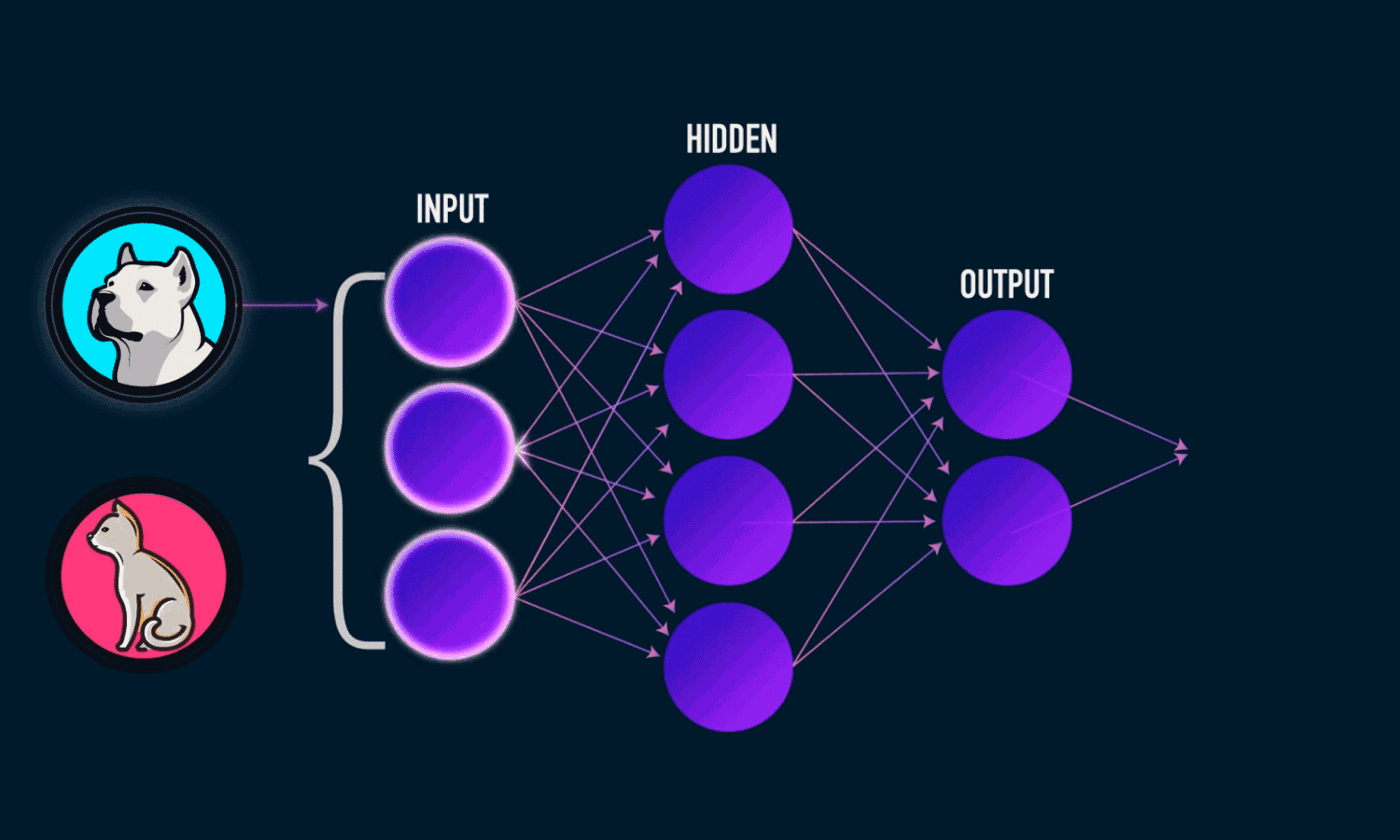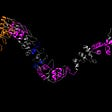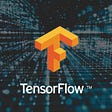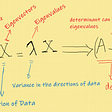# Introduction to Neural Networks

A neural network is a network which closely simulates the learning of a human brain. This is done by using connection weights and biases for each neuron in every layer.

In a neural network, the information is passed to the layer, the layer then computes the weighed sum of the inputs and adds a bias to it and passes it to the next layer in the neural network. This if done for many layers using an appropriate number of neurons in each layer can result in a successful learning of the neural networkA depiction of how the information passes through a neural network

# Structure of a neural network

A neural network has many layers with different or same number of neurons and hence deciding the structure of a neural network can be handy.

Inputs: The inputs to the neural networks should be passed as a matrix of the shape (nx,m) where nx is the number of features in the data and m is the number of training examples in the neural network

And for each layer [l], the weights and biases can be initialized following the following pattern

Weights -> Weights can be initialized as a random matrix with the shape of (n[l], n[l-1]) where n[l] is the number of neurons in the [l]th layer and n[l-1] is the number of neurons in the [l-1]th layer.

Biases -> The biases in the layer can be initialized as a random column matrix with the shape of (n[l], 1) where n[l] is the number of nodes in the [l]th layer

# Activation functions

Just calculating the weighted sum is not enough for a neural network to learn and hence we need to use some kind of non linear function which transforms the input so that it can be fed in the next layer. For this purpose, we have many activation functions

1. Sigmoid function

This activation function is the activation function which is also used in logistic regression. This activation function has the following properties

1. The value of the outputs of this function is always in the range of (0,1) for any input
2. The value of function at 0 is 0.5 , so 0.5 can be used as a classification threshold for two class classification, where the inputs whose sigmoid value is less than 0.5 can be classified as one category and the inputs whose sigmoid value greater than 0.5 can be classified as another category

The problem with sigmoid function is that its output becomes 1 at around an input value of 5 and conversely it becomes 0 at an input value of -5 and the gradient of the function becomes 0 which means that the gradient descent algorithm stops learning, hence we come to the second activation function

2. Tanh function

This activation function has the following properties

1. It has an output value of -1 to 1 for any input value
2. It is centered at 0 as the value at x=0 is 0

Benefits of tanh activation function

1. Most values are very close to -1 or 1 which reduces the burden of multiplying numbers
2. This is centered around 0 which further makes the calculations easier
3. It standardizes the inputs and converts them from (-1,1) with mean = 0

Demerits of tanh — Just like what we observed with sigmoid, it is either 1 or -1 and the gradient of the function becomes 0 which means that the gradient descent algorithm stops learning, hence we come to the third activation function

3. Rectified Linear Unit function

Properties of Rectified Linear Unit function

1. The output for all negative inputs is 0
2. The slope of the output for all positive inputs is 1

In this function we can see that the output for all the negative values becomes 0. The main advantage of Rectified linear unit is that the gradient does not become 0 and the gradient descent algorithm does not stop learning

The peculiarity of Rectified Linear Unit is that the output for all the negative inputs becomes 0 as we kind of ignore it while training the network which may not be desirable

4. Leaky Rectified Linear Unit function

Properties of Leaky Rectified Linear Unit function

1. The output for all negative inputs is a
2. The slope of the output for all positive inputs is 1

The main difference between the Leaky Rectified Linear unit function and Rectified Linear unit function is that the negative inputs are made 0 in the Rectified Linear unit function and they are diminished by multiplying the negative inputs by a (usually 0.01) in Leaky Rectified Linear unit function which kind of considers the negative inputs also but reduces their weightage in the model

5. Softmax function

The softmax activation function is used in the output layer in case of multi class classification because of the following properties

1. The outputs of the softmax function are in the range (0,1)
2. The outputs of the softmax function sum up to 1

Because of these two properties, the output of the softmax function can be interpreted as the probabilities for the input belonging to that particular class.

# Forward Propagation in Neural Network

Forward propagation through a neural network can be considered as

For any layer, the forward propagation output can be calculated as

The output of the network can be considered as the output of the last layer of the network

Where a is the output of the layer and g is the activation function for that layer

# Back Propagation

Back propagating through a neural network implies learning from the errors that the network made and re adjusting the weights and biases in order to reduce the errors of the network

In case of softmax activation function in output layer, the outputs should be one hot encoded in order to perform back propagation

# Training the neural network

In order to train a neural network, first we need to perform forward propagation, then we need to calculate the changes in weights and biases for the neural network and then we need to update the weights and biases in order to improve them. We usually perform the training for many iterations in order to obtain the best possible parameters

Training a 4 layer neural network

Step 1 — Perform forward propagation

Step 2 — Backward Propagation

Step 3 — Update weights and biases

Python implementation of a neural network from scratch

Written by:

Aayushmaan Jain

Github Profile

Website

--

--

--

A data science enthusiast currently doing bachelor's degree in data science

Love podcasts or audiobooks? Learn on the go with our new app.

## What happened when a neural network was tasked with predicting the price of Shampoo?## Predicting Flu Vaccinations (PROJECT NOTS)## Intro to CASP for Machine Learning Researchers## Practical Computer Vision using OpenCV and Python (Basics)## Introduction To Natural Language Processing## Lane detection for ADAS application with sliding windows technics## Natural Language Processing## Abstractive Text Summarization with NLP## Aayushmaan Jain

A data science enthusiast currently doing bachelor's degree in data science

## Naive Bayes Classifier Part-1 (mathematical approach)## Linear Regression using Tensorflow## Exploring Singular Value Decomposition(SVD) from scratch in python## A Technical Review of PCA and its Applications: Calculating Similarity of Two Datasets